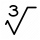# Inverse relationship + cube - math problems

#### Number of problems found: 2

• One third powerWhich equation justifies why ten to the one-third power equals the cube root of ten?
• SeawaterSeawater has a density of 1025 kg/m3, ice 920 kg/m3. 8 liters of seawater froze and created a cube. Calculate the size of the cube edge.

We apologize, but in this category are not a lot of examples.
Do you have an exciting math question or word problem that you can't solve? Ask a question or post a math problem, and we can try to solve it.

We will send a solution to your e-mail address. Solved examples are also published here. Please enter the e-mail correctly and check whether you don't have a full mailbox.

Inverse relationship - math problems. Cube Problems.